Minimum_bounding_circle
import matplotlib.pyplot as plt
import matplotlib.collections as mplc
import libpysal as ps
from shapely import geometry as sgeom
import descartes as des
import pointpats
%matplotlib inline

data = ps.io.open(ps.examples.get_path('columbus.shp')).read()
chains = [chain.parts for chain in data]

points = chains
points

[(8.624129295349121, 14.236980438232422),
(8.559700012207031, 14.742449760437012),
(8.809452056884766, 14.734430313110352),
(8.808412551879883, 14.636520385742188),
(8.919304847717285, 14.638500213623047),
(9.087138175964355, 14.63049030303955),
(9.09996509552002, 14.244830131530762),
(9.015047073364258, 14.241840362548828),
(9.008951187133789, 13.995059967041016),
(8.818140029907227, 14.002050399780273),
(8.653305053710938, 14.008090019226074),
(8.642902374267578, 14.089710235595703),
(8.63259220123291, 14.1705904006958),
(8.625825881958008, 14.22367000579834),
(8.624129295349121, 14.236980438232422)]

Let's plot that polygon by interpreting it in Shapely and using its draw behavior.

poly = sgeom.Polygon(points)
poly


Nifty. Now, I've implemented Skyum's method for finding the Minimum Bounding Circle for a set of points in centrography.

Right now, there's some extra printing. Essentially, if you have sufficiently straight lines on the boundary, the equations for the circumcenter of the tuple $(p,q,r)$ explodes. Thus, I test if $\angle (p,q,r)$ identifies a circle whose diameter is $(p,r)$ or $(p,q)$. There are two triplets of straight enough lines, so their circle equations are modified, and I retain printing for bug diagnostics.

(radius, center), inset, removed, constraints = pointpats.skyum(points)
#p,q,r = cent.skyum(points)
#mbc = cent._circle(points[p], points[q], points[r])
#mbc = cent._circle()

fig = plt.figure(figsize=(10,10))
ax.set_xlim(8, 10)
ax.set_ylim(13,16)
ax.plot([p for p in points], [p[-1] for p in points], 'r')
chull = pointpats.hull(points)
ax.plot([p for p in chull], [p[-1] for p in chull], 'm')
ax.plot([p for p in constraints], [p[-1] for p in constraints], '^b')
ax.plot([p for p in inset], [p[-1] for p in inset], 'ob')
ax.plot([p for p in removed], [p[-1] for p in removed], 'xb')
plt.show()### Cool. How fast is this?

import time

def demo_mbc(chains):
for cidx, chain in enumerate(chains):
points = chain
start = time.time()
(radius, center), inset, removed, constraints = pointpats.skyum(chain)
elapsed = time.time() - start
fig = plt.figure(figsize=(8,8))
parray = ps.common.np.array(points)
ax.set_xlim(parray[:,0].min()*.98, parray[:,0].max()*1.02)
ax.set_ylim(parray[:,1].min()*.98, parray[:,1].max()*1.02)
ax.plot([p for p in points], [p[-1] for p in points], 'r')
chull = pointpats.hull(points)
#ax.plot([p for p in chull], [p[-1] for p in chull], '--m')
ax.plot([p for p in constraints], [p[-1] for p in constraints], '^b')
#ax.plot([p for p in inset], [p[-1] for p in inset], 'ob')
ax.plot([p for p in removed[:-1]], [p for p in removed[:-1]], 'xc')
ax.plot(removed[-1], removed[-1], '*k')
plt.title('Shape #{}, Elapsed Time: {}'.format(cidx, elapsed))
#print(removed)
nonboundary = [p for p in chull.tolist() if p not in constraints]
succeeded = [mbc_poly.contains(sgeom.Point(p)) for p in nonboundary]
for i,v in enumerate(succeeded):
print("Point {i}: {tf}".format(i=i, tf=v))
if not v:
ax.plot(chull.tolist()[i], chull.tolist()[i], 'gH')
plt.show()
plt.clf()

demo_mbc(chains)

Point 0: True
Point 1: True
Point 2: True
Point 3: True
Point 4: True
Point 5: TruePoint 0: True
Point 1: True
Point 2: True
Point 3: True
Point 4: True
Point 5: True
Point 6: True
Point 7: True
Point 8: True

<Figure size 432x288 with 0 Axes>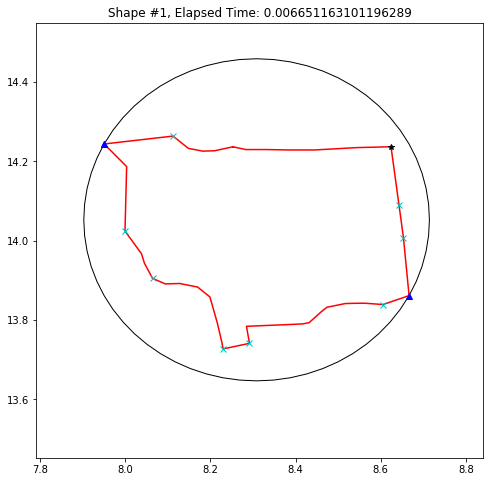Point 0: False
Point 1: True
Point 2: True
Point 3: True
Point 4: True

<Figure size 432x288 with 0 Axes>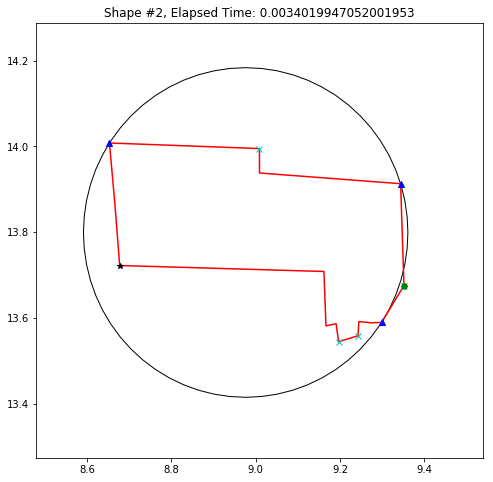Point 0: True
Point 1: True
Point 2: True
Point 3: True
Point 4: True
Point 5: True
Point 6: True
Point 7: True

<Figure size 432x288 with 0 Axes>Point 0: False
Point 1: True
Point 2: True
Point 3: True
Point 4: True

<Figure size 432x288 with 0 Axes>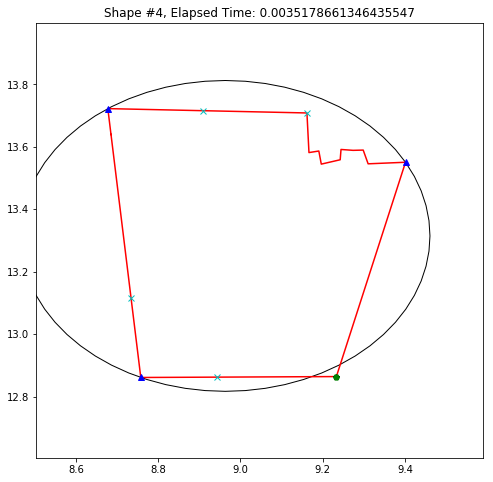Point 0: True
Point 1: True
Point 2: True
Point 3: True
Point 4: True
Point 5: True
Point 6: True
Point 7: True

<Figure size 432x288 with 0 Axes>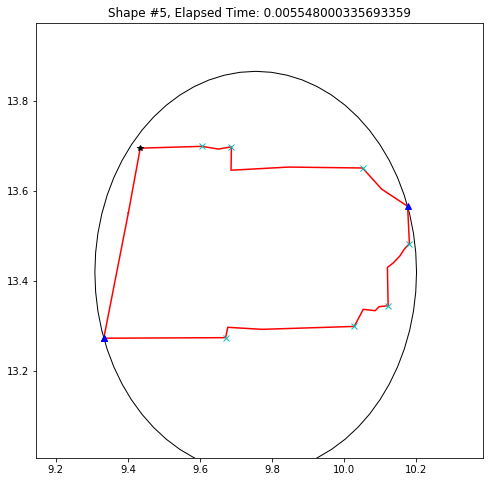Point 0: True
Point 1: True
Point 2: True
Point 3: True
Point 4: True
Point 5: True
Point 6: True
Point 7: True

<Figure size 432x288 with 0 Axes>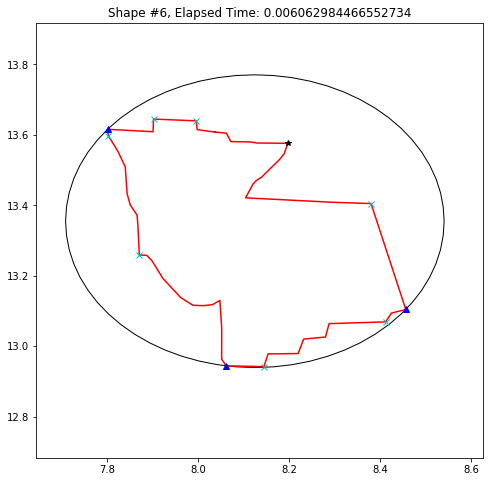Point 0: True
Point 1: True
Point 2: True
Point 3: True
Point 4: True
Point 5: True
Point 6: True
Point 7: True

<Figure size 432x288 with 0 Axes>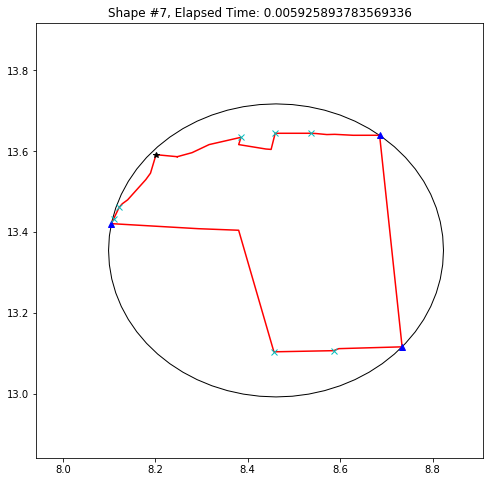Point 0: True
Point 1: True
Point 2: True
Point 3: True
Point 4: True
Point 5: True
Point 6: True
Point 7: True
Point 8: True
Point 9: True

<Figure size 432x288 with 0 Axes>Point 0: True
Point 1: True
Point 2: True
Point 3: True
Point 4: True
Point 5: True
Point 6: True
Point 7: True
Point 8: True
Point 9: True

<Figure size 432x288 with 0 Axes>Point 0: True
Point 1: True
Point 2: True
Point 3: True

<Figure size 432x288 with 0 Axes>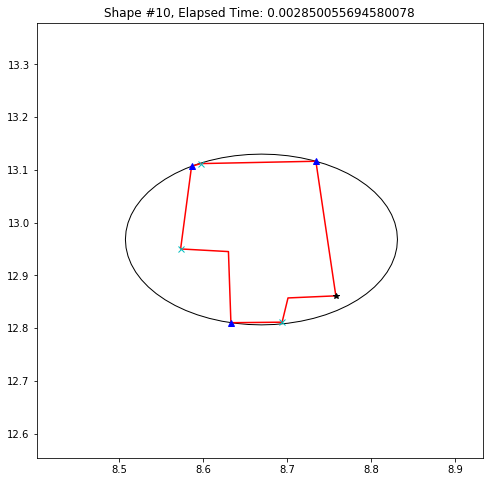Point 0: True
Point 1: True
Point 2: True
Point 3: True
Point 4: True

<Figure size 432x288 with 0 Axes>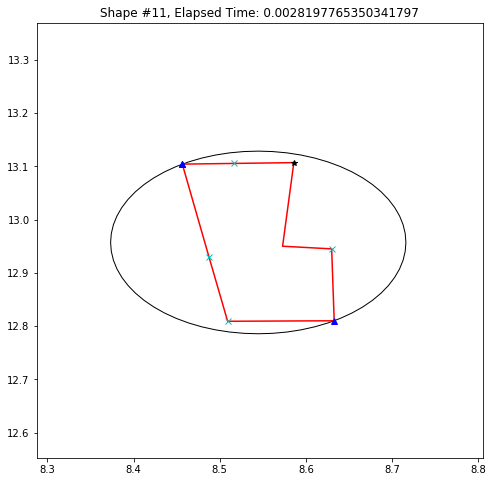Point 0: True
Point 1: True

<Figure size 432x288 with 0 Axes>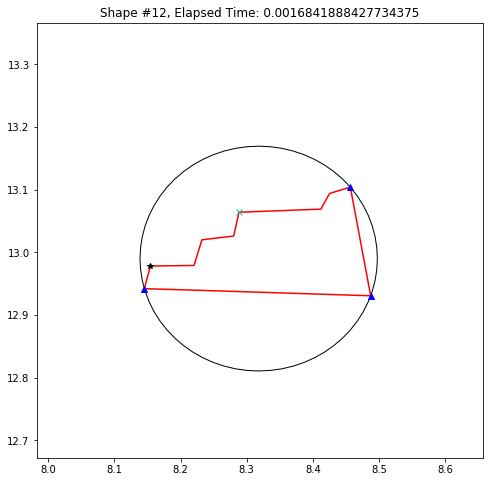Point 0: True
Point 1: True
Point 2: True
Point 3: True
Point 4: True

<Figure size 432x288 with 0 Axes>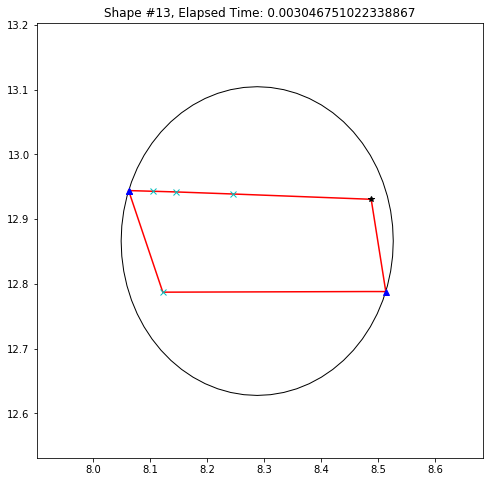Point 0: True
Point 1: True
Point 2: True
Point 3: True
Point 4: True
Point 5: True

<Figure size 432x288 with 0 Axes>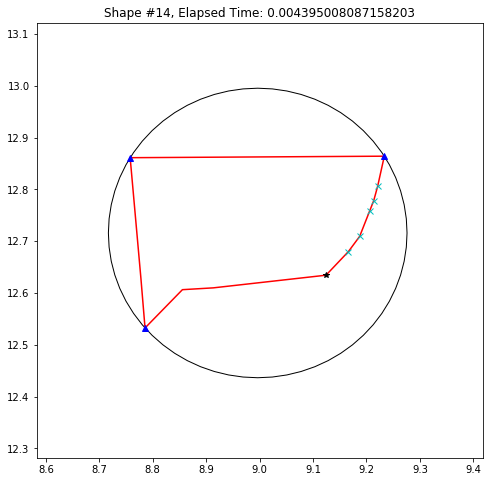Point 0: True
Point 1: True
Point 2: True
Point 3: True
Point 4: True
Point 5: True
Point 6: True
Point 7: True

<Figure size 432x288 with 0 Axes>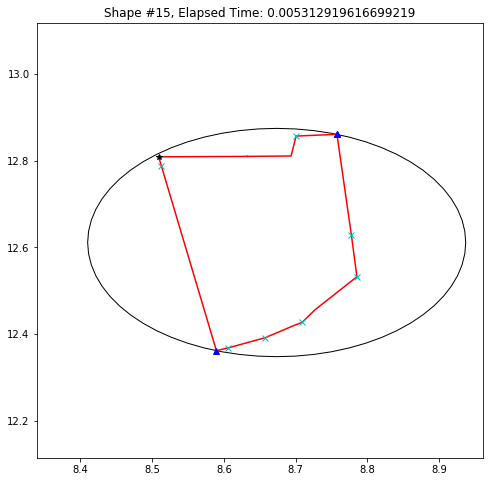Point 0: True
Point 1: True
Point 2: True
Point 3: True
Point 4: True
Point 5: True

<Figure size 432x288 with 0 Axes>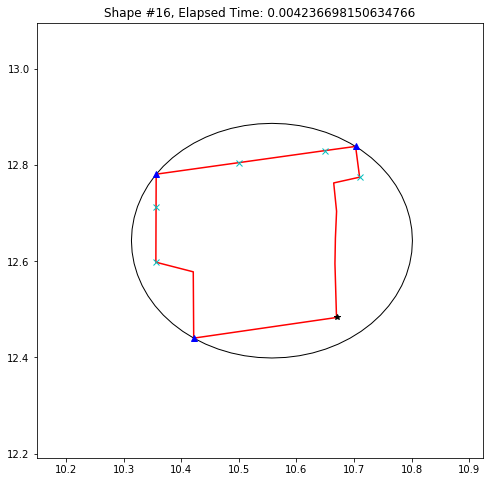Point 0: False
Point 1: False
Point 2: False
Point 3: False
Point 4: False
Point 5: False
Point 6: True
Point 7: False
Point 8: False

<Figure size 432x288 with 0 Axes>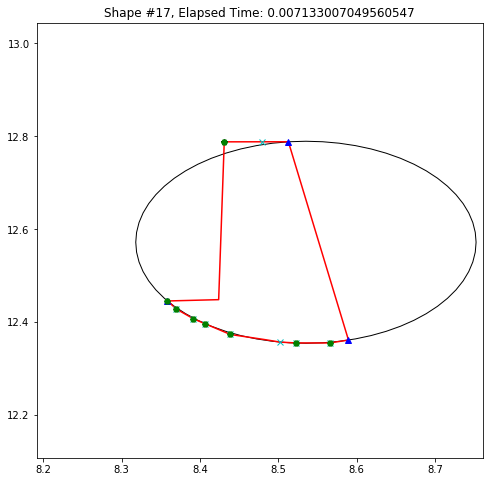Point 0: True
Point 1: True
Point 2: True

<Figure size 432x288 with 0 Axes>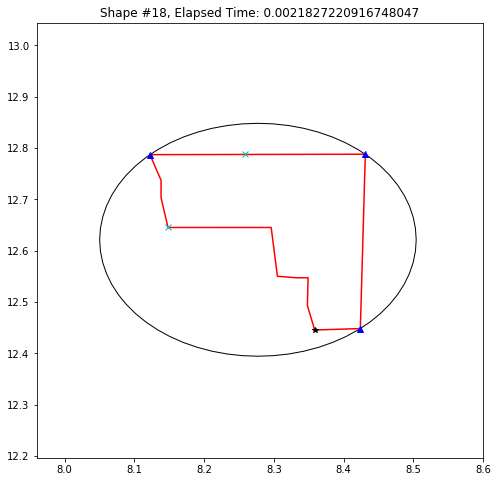Point 0: True
Point 1: True
Point 2: True
Point 3: True
Point 4: True
Point 5: True

<Figure size 432x288 with 0 Axes>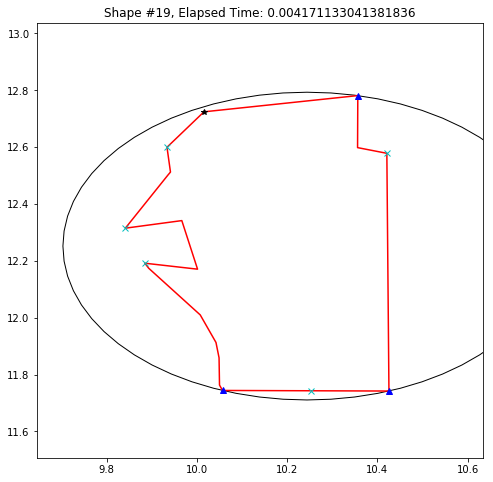Point 0: True
Point 1: True
Point 2: True
Point 3: True
Point 4: True
Point 5: True
Point 6: True
Point 7: True
Point 8: False
Point 9: False
Point 10: False
Point 11: True
Point 12: True
Point 13: True
Point 14: True
Point 15: True
Point 16: True

<Figure size 432x288 with 0 Axes>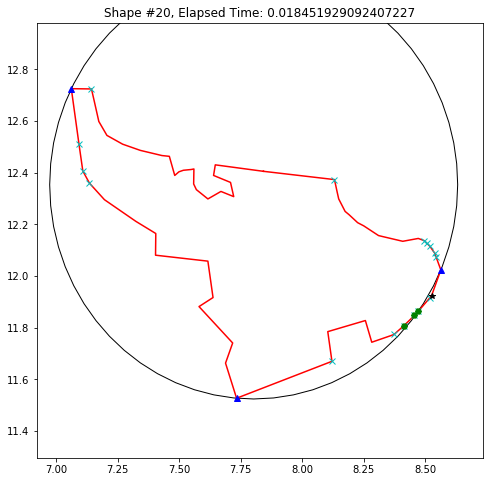Point 0: True
Point 1: True
Point 2: True
Point 3: True
Point 4: True
Point 5: True
Point 6: True
Point 7: True

<Figure size 432x288 with 0 Axes>Point 0: True
Point 1: True
Point 2: True
Point 3: True
Point 4: True
Point 5: True

<Figure size 432x288 with 0 Axes>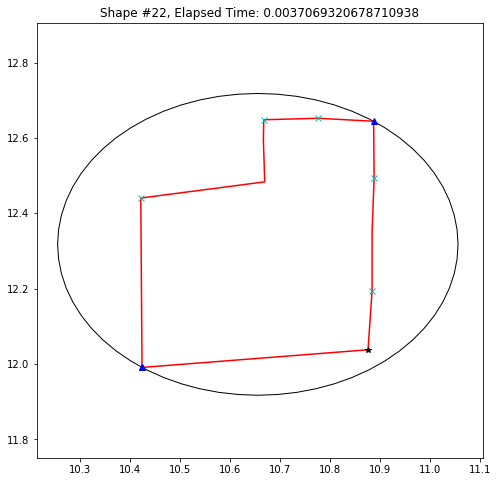Point 0: True
Point 1: True
Point 2: True
Point 3: True
Point 4: True
Point 5: True
Point 6: True
Point 7: True
Point 8: True
Point 9: True
Point 10: True
Point 11: True

<Figure size 432x288 with 0 Axes>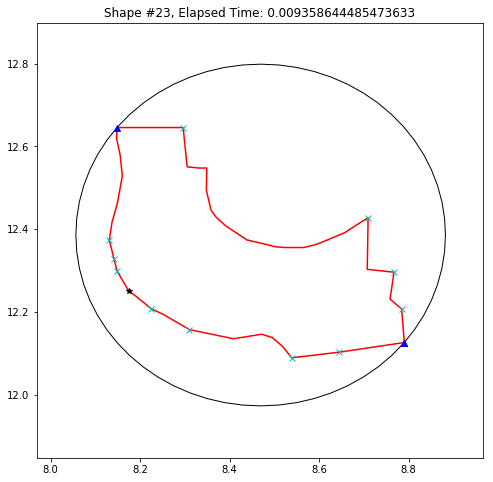Point 0: True
Point 1: True
Point 2: True
Point 3: True
Point 4: True
Point 5: True
Point 6: True
Point 7: True
Point 8: True
Point 9: True

<Figure size 432x288 with 0 Axes>Point 0: True
Point 1: True
Point 2: True
Point 3: True

<Figure size 432x288 with 0 Axes>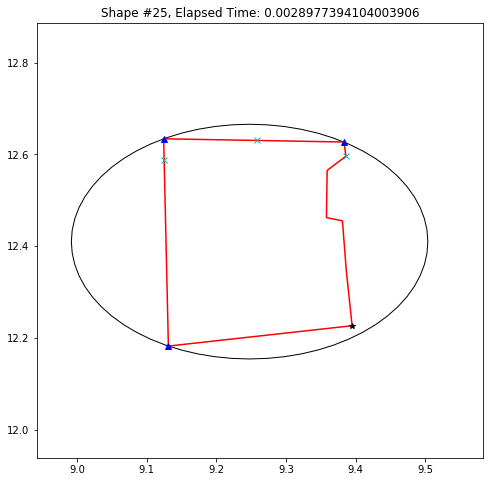Point 0: True
Point 1: True
Point 2: True
Point 3: True

<Figure size 432x288 with 0 Axes>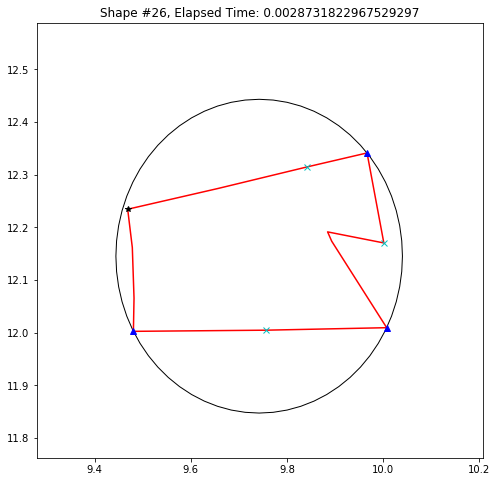Point 0: True
Point 1: True
Point 2: True
Point 3: True
Point 4: True
Point 5: True
Point 6: True
Point 7: True
Point 8: True
Point 9: True

<Figure size 432x288 with 0 Axes>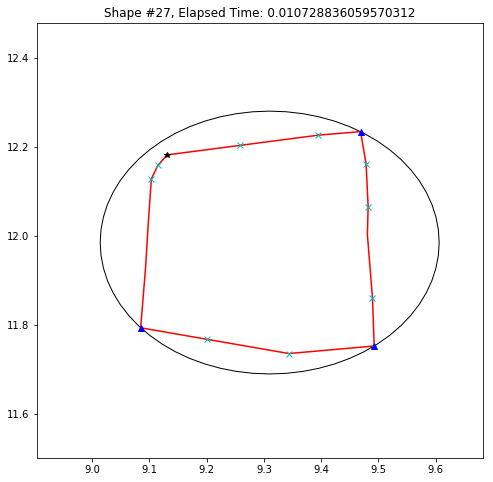Point 0: True
Point 1: True
Point 2: True

<Figure size 432x288 with 0 Axes>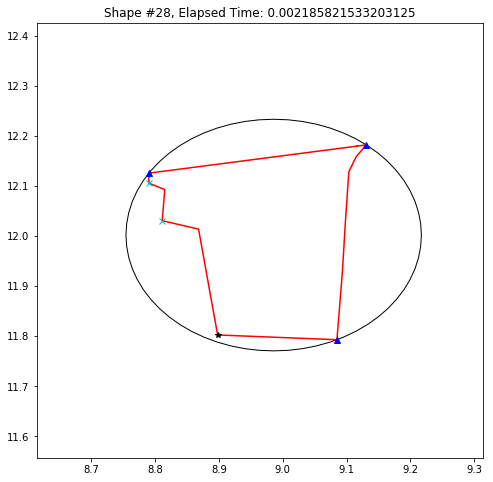Point 0: True
Point 1: True
Point 2: True

<Figure size 432x288 with 0 Axes>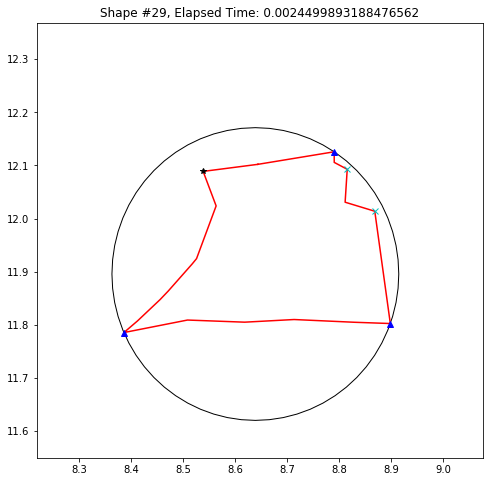Point 0: True
Point 1: True
Point 2: True
Point 3: True
Point 4: True
Point 5: True
Point 6: True

<Figure size 432x288 with 0 Axes>Point 0: True
Point 1: True
Point 2: True
Point 3: True
Point 4: True

<Figure size 432x288 with 0 Axes>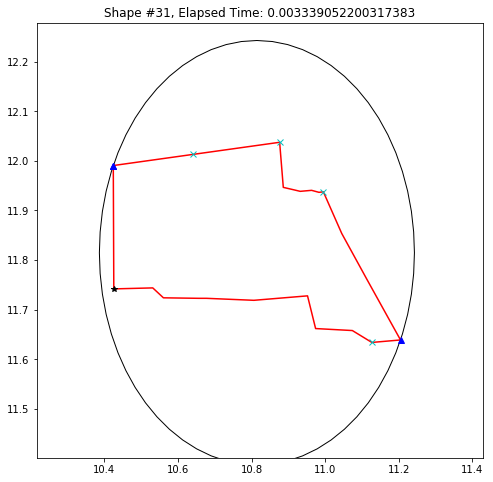Point 0: True
Point 1: True
Point 2: True
Point 3: True
Point 4: True
Point 5: True
Point 6: True
Point 7: True

<Figure size 432x288 with 0 Axes>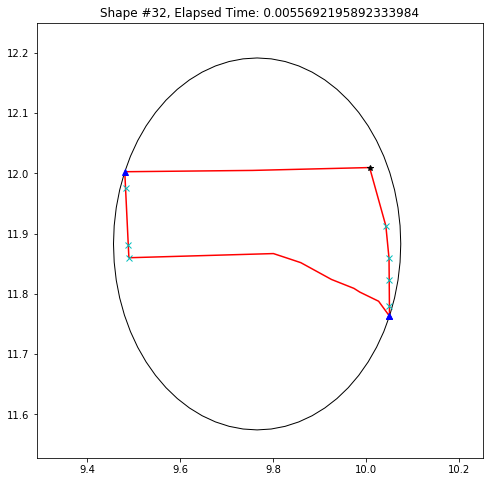Point 0: True
Point 1: True
Point 2: True
Point 3: True
Point 4: True
Point 5: True
Point 6: True
Point 7: True
Point 8: True

<Figure size 432x288 with 0 Axes>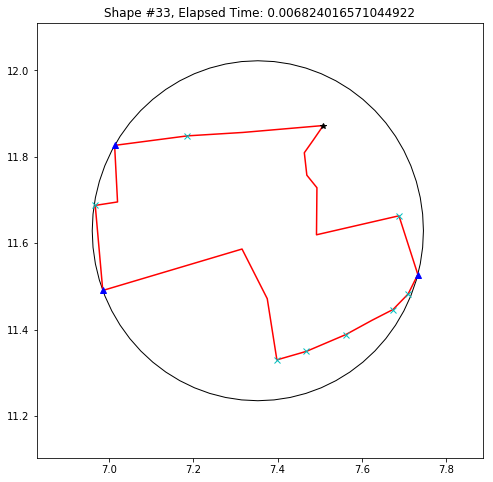Point 0: True
Point 1: True
Point 2: True
Point 3: True
Point 4: True
Point 5: True
Point 6: True
Point 7: True
Point 8: True

<Figure size 432x288 with 0 Axes>Point 0: True
Point 1: True
Point 2: True
Point 3: True
Point 4: True

<Figure size 432x288 with 0 Axes>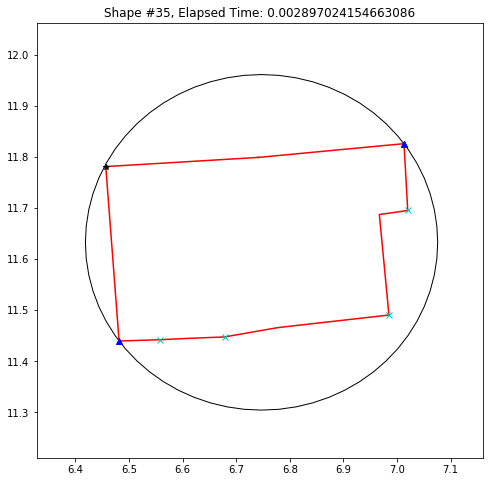Point 0: True
Point 1: True
Point 2: True
Point 3: True
Point 4: True

<Figure size 432x288 with 0 Axes>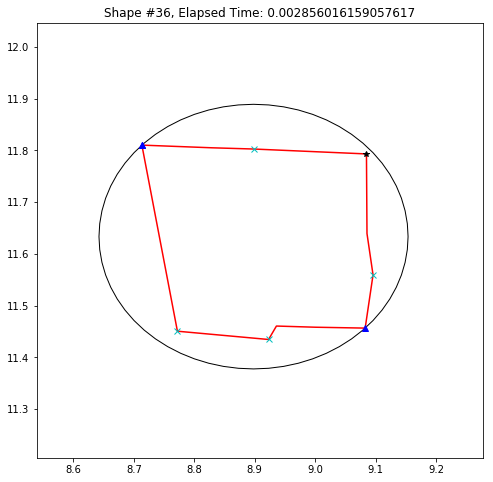Point 0: True
Point 1: True
Point 2: True
Point 3: True

<Figure size 432x288 with 0 Axes>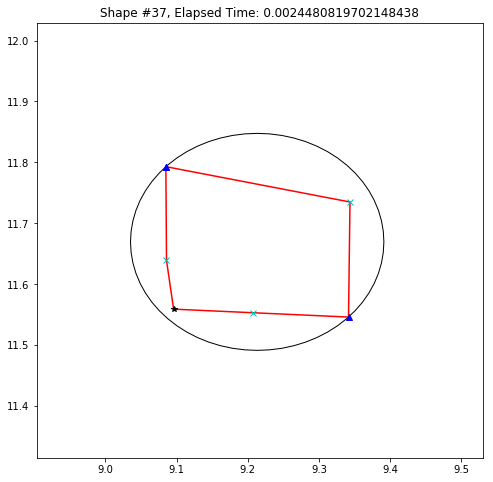Point 0: True
Point 1: True

<Figure size 432x288 with 0 Axes>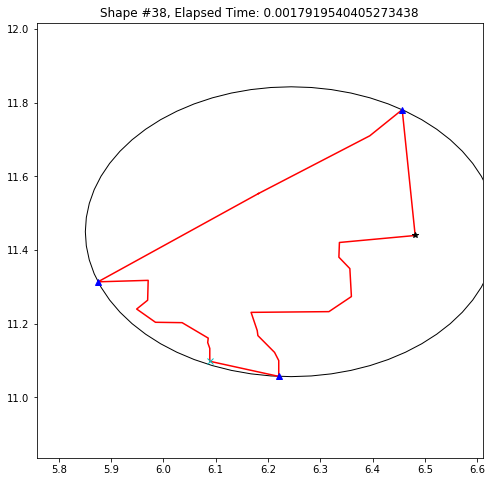Point 0: True
Point 1: True
Point 2: True
Point 3: True
Point 4: True
Point 5: True
Point 6: True
Point 7: True
Point 8: True
Point 9: True

<Figure size 432x288 with 0 Axes>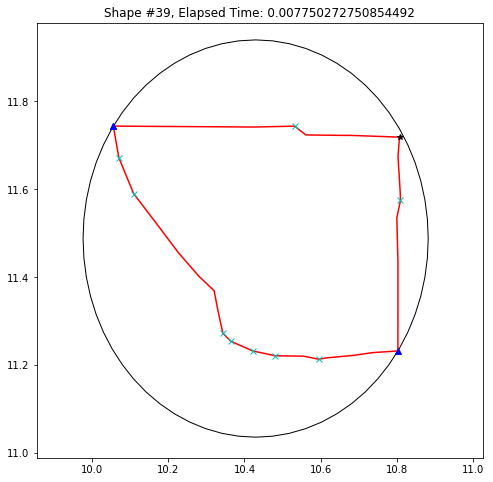Point 0: True
Point 1: True
Point 2: True
Point 3: True
Point 4: True
Point 5: True
Point 6: True
Point 7: True
Point 8: True
Point 9: True
Point 10: True

<Figure size 432x288 with 0 Axes>Point 0: True
Point 1: True
Point 2: True
Point 3: True
Point 4: True
Point 5: True
Point 6: True

<Figure size 432x288 with 0 Axes>Point 0: True
Point 1: True
Point 2: True

<Figure size 432x288 with 0 Axes>Point 0: True
Point 1: True
Point 2: True
Point 3: True

<Figure size 432x288 with 0 Axes>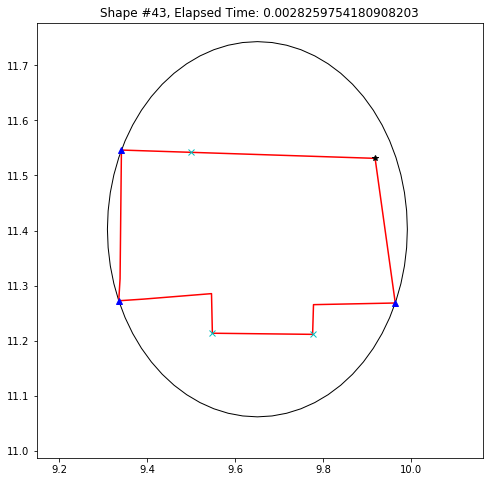Point 0: True
Point 1: True
Point 2: True
Point 3: True
Point 4: True
Point 5: True
Point 6: True
Point 7: True

<Figure size 432x288 with 0 Axes>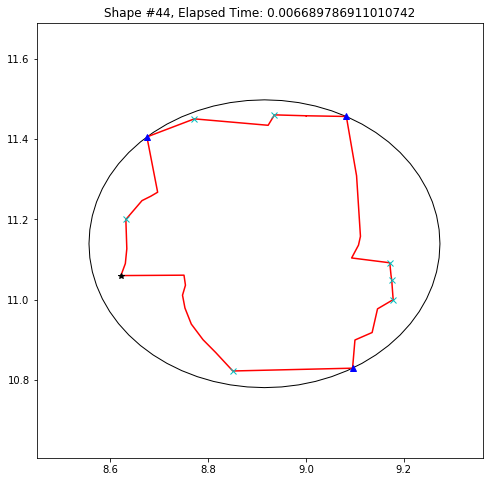Point 0: False
Point 1: True
Point 2: True
Point 3: True
Point 4: False

<Figure size 432x288 with 0 Axes>Point 0: True
Point 1: True
Point 2: True
Point 3: True
Point 4: True
Point 5: True
Point 6: True
Point 7: True

<Figure size 432x288 with 0 Axes>Point 0: True
Point 1: True
Point 2: True
Point 3: True
Point 4: True
Point 5: True
Point 6: True
Point 7: True
Point 8: True
Point 9: True

<Figure size 432x288 with 0 Axes>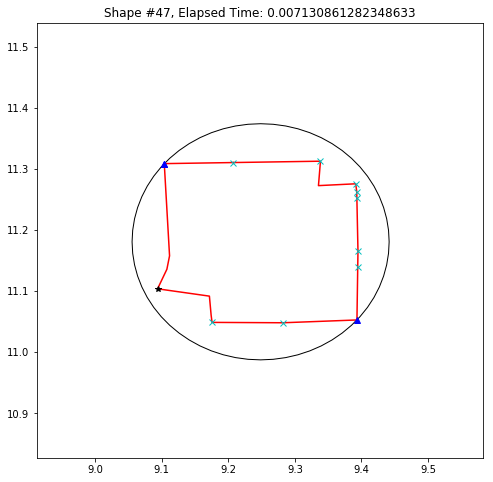Point 0: True
Point 1: True
Point 2: True
Point 3: True
Point 4: True
Point 5: True

<Figure size 432x288 with 0 Axes>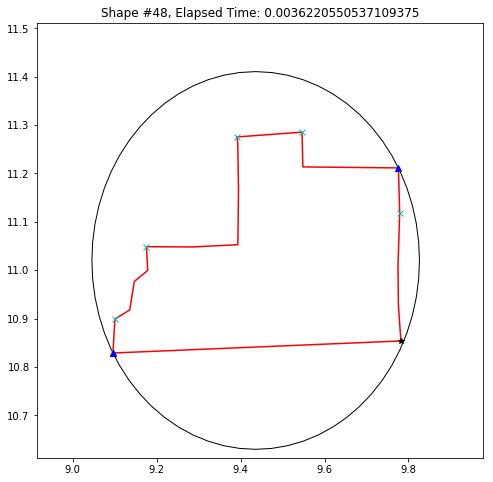<Figure size 432x288 with 0 Axes>
pointpats.hull(chains)

array([[ 9.33329678, 13.27241993],
[ 9.12427711, 12.63424015],
[ 9.3860054 , 12.59624004],
[ 9.47149754, 12.5957098 ],
[ 9.55582809, 12.59519005],
[ 9.72367477, 12.59519958],
[10.01543999, 12.72404957],
[10.09543037, 12.87689972],
[10.09350967, 12.90021992],
[10.08250999, 13.03376961],
[10.02766991, 13.29854012],
[ 9.67700958, 13.29658985]])
plt.plot(*pointpats.hull(chains).T.tolist())
plt.plot(*pointpats.hull(chains).T.tolist(), markerfacecolor='k', marker='o')
plt.plot(*pointpats.hull(chains).T.tolist(), markerfacecolor='k', marker='o')
plt.plot(*pointpats.hull(chains).T.tolist(), markerfacecolor='k', marker='o')

[<matplotlib.lines.Line2D at 0x1b2456d710>]pointpats._circle(chains[-5], chains[-4], chains[-3])

(0.914822771306765, (8.333136366132898, 13.026633376705332))